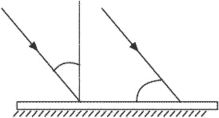# angle of incidence

Also found in: Dictionary, Thesaurus, Medical, Acronyms, Wikipedia.

## angle of incidence

[′aŋ·gəl əv ′in·sə·dəns]
(optics)
The angle formed by a ray arriving at a surface and the perpendicular to that surface at the point of arrival. Also known as incidence angle.
McGraw-Hill Dictionary of Scientific & Technical Terms, 6E, Copyright © 2003 by The McGraw-Hill Companies, Inc.

## Angle of incidence

In terms of solar energy, the angle that the sun’s rays make with an imaginary line perpendicular to a surface. The angle of incidence determines the intensity of the energy that any surface experiences.

## angle of incidenceIncident rays on a surface. Two ways of measuring angle of incidence.
i. The acute angle formed between the chord line of an airfoil and the longitudinal axis of the aircraft on which it is mounted. This is the angle at which the airfoil is attached to an aircraft fuselage when the aircraft is in rigging position. Unlike the angle of attack, the angle of incidence is a fixed entity.
ii. The angle at which a ray of energy impinges upon a surface, usually measured between the direction of propagation of the energy and the perpendicular to the surface at the point of impingement, or incidence. In some cases involving radio waves, the angle of incidence is measured relative to the surface.
References in periodicals archive ?
Figure 5(a) demonstrates the ratio of fields at closest points of both spheres situated one over another at one vertical and illuminated at the angle of incidence of 70[degrees].
It is also interesting to note that the width of the gap in the ternary structure for TE wave is sensitive to the angle of incidence and increases as the incidence angle increases, while the left edge of the band gap is nearly invariant.
The following results show how diffraction efficiency varies with angle of incidence for individual holographic focussing elements, recorded in photopolymer on plastic substrates, as well as a combined stack of three elements.
When the applied wave is given some angle of incidence, the phase matching condition changes.
The reflectance bandwidth for the TE wave (as shown in Figure 4(a)) is obviously enlarged when the angle of incidence increases, as compared to the reflectance bandwidth of normal incidence.
The frequency intervals with negative value of GD and GS are observed only for asymmetric resonators and always located near the resonance frequency or angle of incidence (Figures 2(a)-(b), line 3).
We can see in the graphs (figure 9) pressure coefficient variation ([C.sub.l]) reported at airfoil chord depending angle of incidence ([alpha]) at Re = 272000 for minimum speed of 36 km/h.
In this status, the dimensions are definite; the angle of incidence is zero; only the tensors {[[epsilon].sub.11], [[epsilon].sub.22], [[epsilon].sub.33]} & [[mu].sub.11], [[mu].sub.22], [[mu].sub.33]} change.
The behaviour of reflected and transmitted amplitude ratios relative to frequency, angle of incidence, layer thickness, and porosity is observed numerically for a particular model.
If both media are dielectric in nature (i.e., [k.sub.1] = [k.sub.2] = O), then [R.sub.P] becomes zero at a specific angle of incidence [PHI](B), where [R.sub.P] is the reflection coefficient and it refers to waves parallel to plane of incidence.
3, the angle of incidence ([[theta].sub.i]) is calculated by the inner product of the unit direction vector (i) of the incidence photons and the unit normal vector ([[vector].n]), and the angle of refraction ([[theta].sub.r]) is calculated by "Snell's Law".
This invention includes capturing a first image of at least a portion of a document while the at least a portion of the document is subjected to a first electromagnetic radiation from a first angle of incidence, and capturing a second image of at least a portion of the document while the at least a portion of the document is subjected to a second electromagnetic radiation from a second angle of incidence.

Site: Follow: Share:
Open / Close